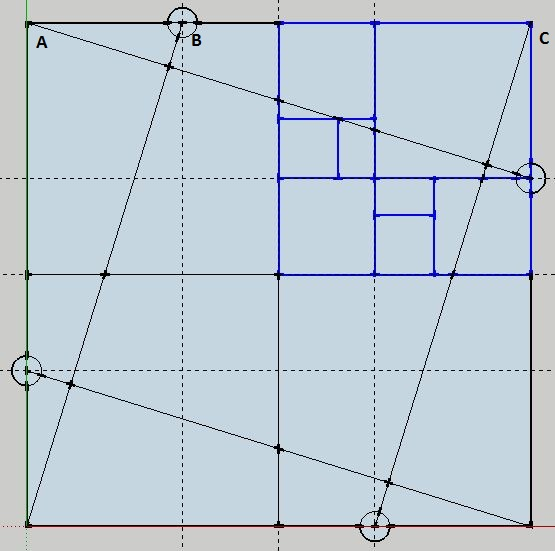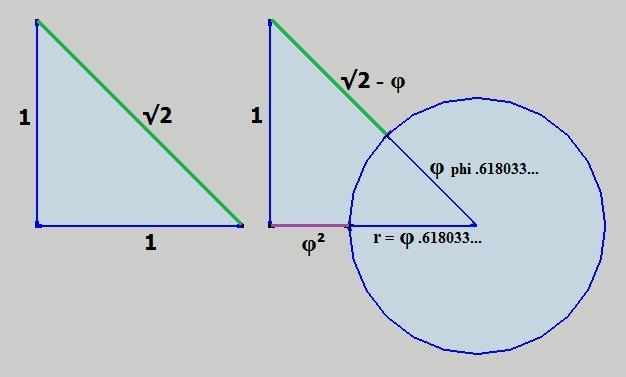Home | Study Links | Poems |

## 48 Golden Ratio SpiralsConstructing golden rectangles begins with this self-explanatory geometric configuration.
Several measurements are given, and the circles and guide lines are the tools required to
progressively reduce the sizes of the golden rectangles.The diagram tilted back and showing the golden rectangle construction starting
in the upper right quadrant. In this diagram there are 8 Golden ratio spirals.The point to which all golden rectangles converge is sometimes called the “Eye of God”.
Here 8 golden rectangles and their converging points form a circle, and it represents
one face of a cube. Therefore, 48 golden spirals signify a sphere within the cube,
and it shows a unique and fixed relationship between this Cube and Sphere ><>
To simplify the idea:Volume Calculations:
Cube 2³ = 8
Sphere 4/3πr³ (r = φ .618...) = .98883923...(V1)

8 / .98883923...(V1) = 8.09029389... x φ .618... = 5.00007660...
And
8 / .98885438...(V2) = 8.09016994... x φ .618... = 5 (has a slightly altered fraction 4/3)

1.6 / ϕ = 0.98885438...(V2) / π / r³ = 1.33335376...(the new "4/3")

This Volume formula represents a free, balanced, and unrestricted Sphere:

V = (√1.25 + 1.5) x 1.6 x r³

V = [ (√1.25 + 1.5) x 1.6 ] or [ π x 1.33335376... ] or [ (1.6 / ϕ) + 3.2 ] x

In this example a balanced Cube and Sphere of equal diameters:
Cube (side = .618... x 2) ³ = V 1.8885438...
Sphere (1.6 / ϕ) = V 0.98885438...

V 1.8885438... / V 0.98885438... = 1.90983005... [ 5 / (ϕ + 1) ]
And 0.98885438... - (1.8885438... / 10) = 0.8
8 / 1.90983005... = 4.18885438... [ (1.6 / ϕ) + 3.2 ]

A Sphere in a Cylinder:
Imagine a cylinder volume πr²h ( r = φ .618... and h = 2 x r ) which contains
a sphere with the common formula 4/3πr³ (r = φ .618...) V = .98883923...
Cylinder V 1.48325884... / Sphere V .98883923... = 1.5 Here the fraction 4/3
reduced the sphere size, so as to accommodate and keep the cylinder volume!

Explanation for the two different Sphere volumes:
Sphere one V1= .98883923... is restricted and the second V2= .98885438... is not.
Sphere one is of slightly lesser volume because it is surrounded and "compressed"
within a "Cube", whereas the second sphere in a free state is not. Imagine a sphere
within a cube of equal diameters, where one or the other has to give up space.
So, the formula V = (√1.25 + 1.5) x 1.6 x r³ consisting of true numbers pi and phi,
represents spheres that exist in a perfectly harmonious, free and balanced state.

I have come to realize that measurements and ratios occurring in geometric drawings,
show that there is one arithmetical number that is prominent, and that number is 5.
In Biblical scripture the number 5 symbolizes "Grace".